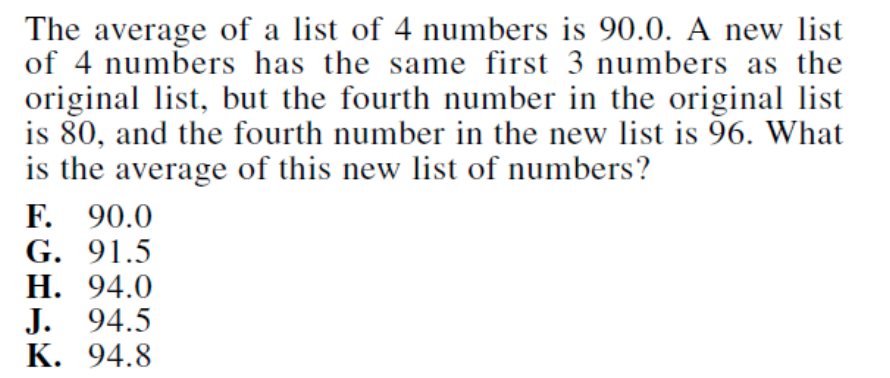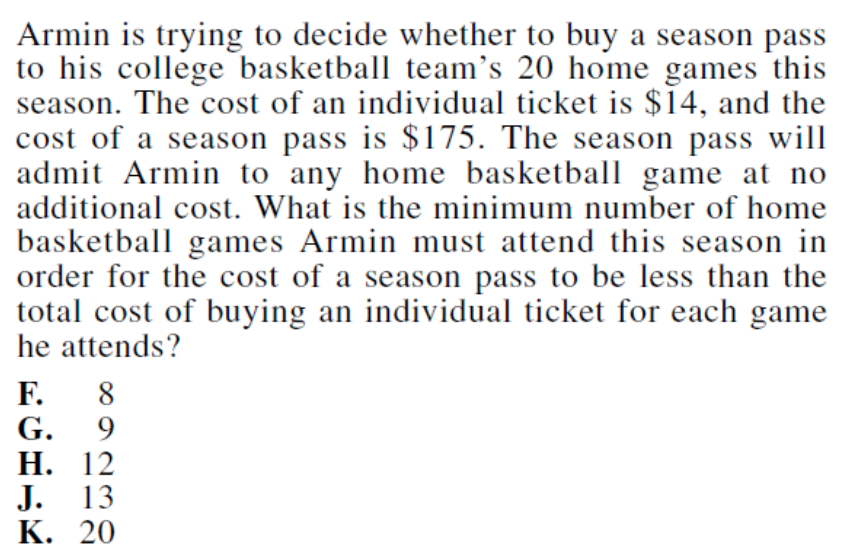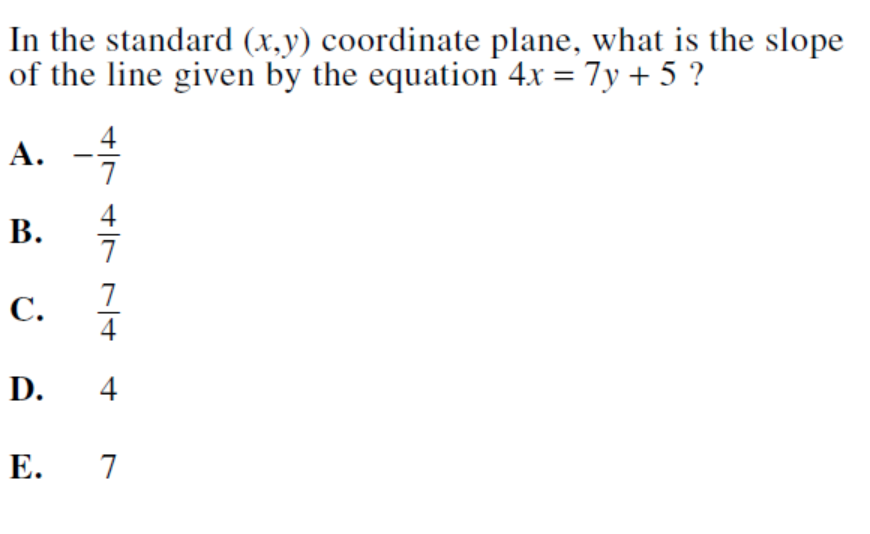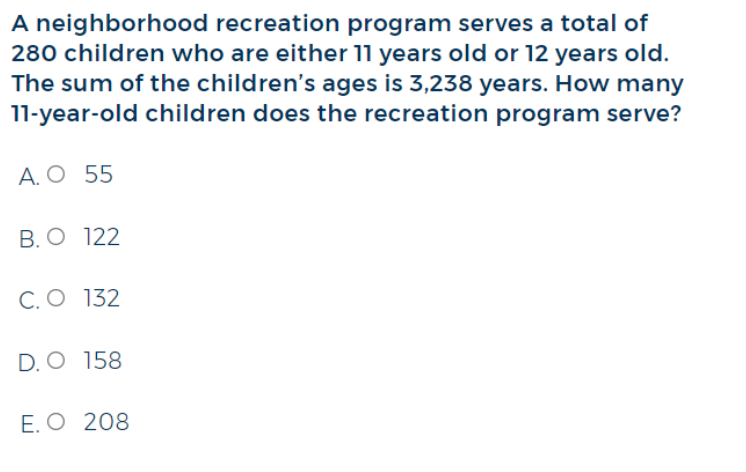### What are your chances of acceptance?

Duke University
UCLA
Unweighted GPA: 3.7
1.0
4.0
SAT: 720 math
200
800
| 800 verbal
200
800

#### Extracurriculars

Low accuracy (4 of 18 factors)Thamira Skandakumar
10

# Guide to ACT Math Algebra + Practice Questions

### Do you know how to improve your profile for college applications?

See how your profile ranks among thousands of other students using CollegeVine. Calculate your chances at your dream schools and learn what areas you need to improve right now — it only takes 3 minutes and it's 100% free.

## What’s Covered:

Algebra is something we use almost any time we deal with math. The manipulation of different types of numbers can be difficult, especially if you haven’t familiarized yourself with the various number types. The ACT Math Test will test your ability to maneuver around these types of questions.

In this post, we’ll cover the number and quantity portion of the ACT Math Test and teach you strategies to answer these questions with ease!

## Overview of ACT Math Algebra

The ACT Math Test consists of 60 multiple-choice questions to be answered within 60 minutes. The test assesses math skills that students typically learn up until the beginning of their senior year of high school. A calculator is allowed for the math portion of the ACT, however, all questions are able to be solved without the use of one.

Algebra questions comprise 12-15% of the math test and tests students on their ability to solve, graph and model different types of expressions. Students are expected to be able to manipulate linear, polynomial, radical and exponential relationships as well as finding solutions to systems of equations (this can involve using matrices).

Not only will you be given an overall score for the ACT Math Test, but you will also receive subscores for each of the subsections in the Math test. The ACT also provides the following directions for the math section of the exam which we recommend you become familiar with prior to taking the exam:

## How will the ACT Impact My College Chances?

Your ACT scores, just like SAT scores, will be used by most colleges to determine your academic competitiveness. This means that scoring well on the ACT is important, especially if you want a better chance of being accepted into some of the top colleges across the country.

If you want to know how your ACT scores, along with your GPA, high school classes, and extracurriculars will impact your odds of admission, check out CollegeVine’s FREE chancing engine! This tool will take into consideration the above data to determine your chances of getting into various schools. Our chancing engine will even offer tips to help you improve your profile!## 10 Difficult Algebra Questions

Below are 10 different algebra questions to give you insight as to what these questions look like and different strategies to combat these problems.

### Question 1Algebra questions on the ACT require you to be able to solve word problems. When dealing with word problems, we need to make sure to read carefully so that we don’t misread or miss any information!

From this problem statement we see that the average (mean) of a list of 4 numbers is 90.0. This means that the total sum of four numbers, which we will refer to as x, divided by four, is equal to 90 or 90 = x / 4. We also know that the new average of the new list of numbers equal to the sum of the four values, which we will refer to as z, divided by 4 or z / 4.

We also know that the new list of four numbers has the same first 3 numbers as the original list (but the fourth is different). This means that the sum of the first three numbers is equal in both sets, which we will refer to as y from here on out. Therefore, in the original set, the numerator x is equal to y + 80 while the numerator for the new list z is equal to y + 96.

We can determine what y (the sum of the first three numbers) is by utilizing the equation we determined earlier. If we know that 90 = x / 4 and that x is equal to y + 80 for the original set, we see that:

90 = (y + 80) / 4

360 = y + 80

y = 280

We can then substitute y + 96 into the value of z to find the average of the new list which is z / 4:

(y+96) / 4 = (280 + 96) / 4 = (376) / 4 = 94

Therefore the average of the new list is equal to 94.0.

### Question 2Algebra questions on the ACT also require you to be able to manipulate exponents; make sure you know all the exponential rules! In this type of problem, we could spend time entering the following expression into our calculator to determine what the answer is. But there is a much faster way to solve this by hand!

When dealing with scientific notation and exponents we can realize that we can divide like terms. This means that the first step is to divide 4.8 by 1.6 since they are both decimal numbers:

(4.8) / 1.6 = 3.0

The next thing is to divide the exponents and since both 10-7 and 10-11 have the same base, this is easy to do! We do this by using the quotient rule which states:

(am) / an = am – n

Therefore, (10-7) / 10-11 = 10-7 – (-11) =104

When we combine the two found answers, we see that the solution is 3.0 x 104 .

### Question 3Another important topic to study up on before taking the ACT is easy polynomial factorization rules. For example, in this question, we realize that x3  – 64 is a difference of cubes which takes on the basic form a3 – b3.

A polynomial that is a difference of cubes has the following factorization:

a3 – b3 = (a – b)(a2 + ab + b2)

By equating a3-b3 to x3-64, we see that:

a3 – b3 = x3 – 64

a = x

b = 4

Therefore, when we factor x3 – 64 and equate a to x and b to 4, we see that:

x3 – 64 = (x – 4)(x2 + 4x + 16)

Both (x – 4)and (x2 + 4x + 16) are factors of the polynomial x3 – 64 which means that answer choice A is the correct factor.

### Question 4We are given a system of equations and the first thing when dealing with a system of equations is to find one equation that defines them both.

Given that y = x2 and rx + sy = t we see that:

rx + sx2 = t

sx2 + rx – t=0

We see now that the following equation is a quadratic equation and can easily use the discriminant to help us determine the total real-number coordinates. When the discriminant is greater than 0, there are two real-number coordinates. The discriminant in a quadratic equation with the formula ax2 + bx + c = 0 is equal to b2 – 4ac.

Therefore, in this situation, we see that the discriminant is:

r2 – 4(s)(-t) = r2 + 4st

Anytime this expression is greater than 0, as seen in answer choice F, there will be more than one (x , y) solution.

### Question 5This question requires the ability to analyze inequalities. In this problem, we need to figure out how many home basketball games Armin needs to attend at minimum in order for the cost of the season pass (\$175) to be less than the total cost of buying individual home game tickets (\$14).

If x is equal to the number of games Armin attends, then we need to solve for the point when the season pass is less than the cost of individual games:

14x > 175

x > 12.5

Here we see that the answer needs to be either answer choice H, 12 games, or J, 13 games since x = 12.5. If Armin attends 12 games, the total cost of individual games equates to \$168 and if Armin attends 13 games, the total cost of individual games equates to \$182. Here we see that attending 12 games is cheaper than buying a season pass while attending 13 games is not. Therefore, if Armin attends 13 games at minimum, the cost of a season pass will be less.

## Discover your chances at hundreds of schools

Our free chancing engine takes into account your history, background, test scores, and extracurricular activities to show you your real chances of admission—and how to improve them.

###You can expect to see at least one slope related question on the math portion of the ACT. Slope is defined as rise over run, or the change in y over the change in x. When giving this equation a quick glance, we might assume the slope of the equation to be 4 since that is the value of the coefficient of x. However, y has a coefficient not equal to 1, therefore, this answer choice is incorrect. Therefore, when we are given a linear equation, we need to define the equation in terms of y to know the true slope of the equation:

4x = 7y + 5

7y = 4x – 5

y = (4/7)x – (5/7)

Therefore, the correct slope of this equation is (4/7).

### Question 7This is quite the lengthy word problem as we see above. Let’s break down the problem step by step to find out the difference between the two values.

First, we see that the sum of 2 positive numbers is 151. Let us assume that these 2 positive numbers are x and y:

x + y = 151

We also know that “the lesser number is 19 more than the square root of the greater number.” At first sight, this seems quite a bit of information. One thing to note is that when we see the word is in a word problem, we know that word is synonymous to equals to.

Let’s equate x to be the lesser number (and y the greater number).  Breaking down the word problem we get:

the lesser number (x) is (=) 19 more (+) than the square root of the greater number √y

or

x = 19 + √y

x – √y = 19

Now we have a system of equations in which we can utilize the elimination method to solve for both values:

(x + y = 151) – (x – √y = 19) = (y + √y = 132) = √y (√y + 1) = 132

At first glance, it might be easier to use a calculator to determine what y is equal to. However, if we say that √y = z we can do the following:

z (z + 1) = 132

This suggests that two consecutive numbers, when multiplied, equate to 132. The only possible set of consecutive values are 11 and 12. Therefore, we see that z = 11 which suggests that y = 121 since √y = z.

Going back to our original set of equations, we get:

x + 121 = 151

x = 30

Therefore, the value between the greater number and the lesser number is:

y – x = 121 – 30 = 91

### Question 8Since this is another word problem, we need to read carefully! In this problem, we see that the ABC Book Club charges \$40 for an initial fee and \$2 per book read in that month while the Easy Book Club charges \$35 for an initial fee and \$3 per book read in that month.

To begin, we need to convert these statements into expressions. We can assume that the initial fee for each club is the y-intercept and that the charge per book read in a month equates to the slope. Therefore, if we assume x to be the books read in a month, the equation for the two clubs are as follows:

ABC Book Club: 40 + 2x

Easy Book Club: 35 + 3x

To determine how many books must be read in one month for the total charges to be equal, this means we need to equate the two expressions to one another and solve for x:

40 + 2x = 35 + 3x

x = 5

As shown above, the correct answer is H since 5 books need to have been read in one month for the charges of both clubs to be equal.

### Question 9Here we see that we are given four different equations that all equate to t. To find which of the variables has the greatest value, we need to solve for t for each equation:

1.23w = t w = t / 1.23

1.01x = tx= t / 1.01

0.99y = ty = t / 0.99

0.23z = tz= t / 0.23

Now we see that all these equations can be compared since they are all in terms of t. We also see that the expression for z has the smallest denominator, thereby equating to the greater number. To prove this thought process is right, we convert all the equations to the following:

w = t / 1.23 = 0.81t

x = t / 1.01 = 0.99t

y = t / 0.99 = 1.01t

z = t / 0.23 = 4.35t

### Question 10System of equations are question types that you should become familiar with while studying for the Math portion of the ACT. Practice solving problems using the substitution method as well as the elimination method so that you can determine what method will be the most time-efficient for different problems (we use the elimination method in problem 7).

We are told that there are a total of 280 children who are either 11 years old or 12 years old in the neighborhood recreation program. Let us assume that 11 year olds can be represented by the variable e while twelve year olds can be represented by the variable t. This means that:

e + t = 280

We also know that the sum of the children’s ages is 3238 years. Therefore, the equation that relates to this statement is:

11e + 12t = 3238

We now have a set of equations we can use to solve for the variables e and t.

Since we want to determine how many 11 year olds are in the program, we want to include e in the expression when using the substitution method. This means we can modify the first equation to be:

t = 280 – e

Substituting this into the second equation we get:

11e + 12 (280 – e) = 3238

11e + 3360 – 12e = 3238

e = -122

e = 122

Therefore, there are a total of 122 eleven year olds in the program.

## Final Tips and Strategies

### Learn How to Use Your Calculator

Calculators are allowed for the math portion of the test, which means that it is a good idea to familiarize yourself with your calculator. When taking practice exams or studying up on math problems, you should try practicing with the same calculator that you will be using on test day. You might even be able to learn some useful tricks and shortcuts that will save you plenty of time.

While using a calculator is a good idea, you should be wary of over-relying on your calculator. The ACT Math Test is written so that a calculator is not needed for any problem. For this reason, it may be a good idea to use your calculator only while checking your answers or if you come across a hard problem you don’t know how to solve.

Another trick while taking practice tests is to time yourself while trying to answer a question with and without a calculator. This will help you determine which types of questions may need a calculator and which won’t, thereby maximizing your test-taking time.

### Stuck? Use Logic

If you come across a difficult question, don’t spend too much time trying to solve the problem. If you immediately recognize that the problem is difficult, skip it and come to it later. When you do return to the difficult problem, try to use logic to eliminate answers that are incorrect. You can do this by comparing the answer choices to each other in an attempt to see how they differ. Differences that you notice can provide clues as to what is being asked of the question or whether the answer choice is too absurd. Once you’ve eliminated as many incorrect answers as possible, it is best to make an educated guess from the answer choices remaining.

The ACT Math Test, unlike the SAT, does not give you a set of equations you can use to help answer questions on the exam. Therefore, it is a good idea to become familiarized with basic formulas that will help you complete the exam. Knowing the purpose of a formula as well as when and how it can be used will help decrease the amount of time you spend on a question.

It is crucial that you become familiarized with important formulas before test day so that you are able to practice with them ahead of time. You can visit CollegeVine’s guide on the 31 ACT math formulas you need to know to help you get started!

Check out some of our other posts as you study for the ACT Math Test:Short Bio
My name is Thamira Skandakumar and I am from Vancouver, Washington. I am a current fourth year at UCLA studying Bioengineering with a minor in Electrical Engineering. In the summer, I will be moving out to San Diego to work at Medtronic in their Ventilator division.